# Bluetooth LE Positioning by Using Direction Finding

This example shows you how to calculate the 2-D or 3-D position of a Bluetooth® low energy (LE) node by implementing Bluetooth direction finding features and the triangulation-based location estimation technique by using Bluetooth® Toolbox™. The Bluetooth Core Specification 5.1  introduced angle of arrival (AoA) and angle of departure (AoD) direction finding features to support centimeter-level accuracy in Bluetooth LE location finding.

Using this example, you can:

• Simulate the direction finding packet exchange between the Bluetooth LE node and each locator to estimate the angles between them.

• Estimate the location of Bluetooth LE node by using triangulation-based location estimation technique in an additive white Gaussian noise (AWGN) channel.

• Measure the positioning accuracy of the Bluetooth LE node related to the bit energy-to-noise density ratio (Eb/No).

### Bluetooth LE Localization

Bluetooth technology provides different types of location based services . On a high level, these services can be split into two categories.

• Proximity Solutions: To estimate the distance between two devices, the Bluetooth proximity solutions previously used received signal strength indication (RSSI) measurements.

• Positioning Systems: To estimate the position of device, the Bluetooth positioning systems use trilateration based on several RSSI measurements to estimate the position of the device.

Previous versions of Bluetooth provide only meter-level accuracy in estimating the device location. The Bluetooth Core Specification 5.1  introduced new direction finding features that support centimeter-level accuracy in estimating the location of a device. For more information about direction finding services in Bluetooth LE, see Bluetooth Location and Direction Finding.

#### Direction Finding Methods

Bluetooth direction finding provides two distinct methods each of which exploits the same underlying basis. These direction finding methods are AoA and AoD. Each of these techniques require one of the two communicating devices to have an array of multiple antennas. In the AoA and AoD techniques, the antenna array is present at the receiver and transmitter, respectively.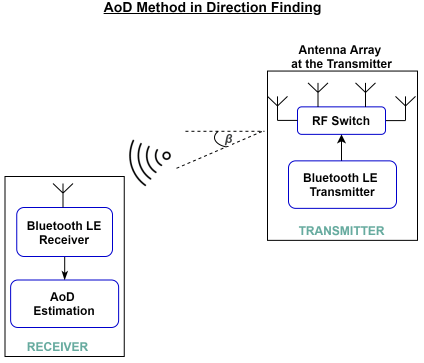#### Antenna Arrays

Use a uniform linear array (ULA) or uniform rectangular array (URA) to calculate the direction of a signal. Simple linear designs like ULAs enable you to calculate only azimuth angle from a signal. Two dimensional arrays like URAs enable you to calculate both the azimuth and elevation angles in the 3-D half space. For more information about antenna arrays, see Bluetooth Location and Direction Finding.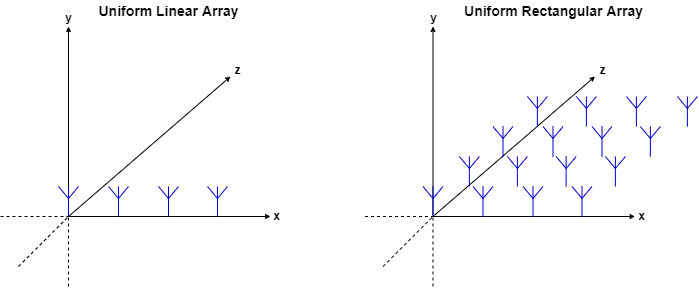Calculating the elevation and azimuth angles of the signal relative to a reference plane is common in these antenna arrays. This figure shows the concept of azimuth and elevation angles.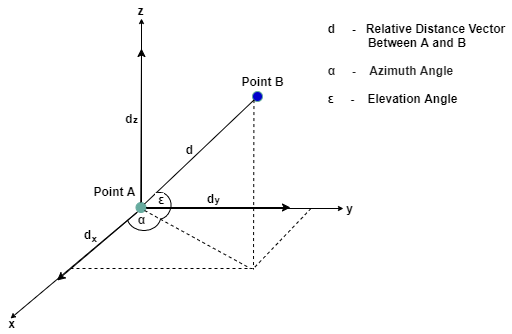• Azimuth angle: This angle is the angle between the x-axis and the orthogonal projection of the vector onto the xy-plane. The angle is positive in going from the x-axis toward the y-axis.

• Elevation angle: This angle is the angle between the vector and its orthogonal projection onto the xy-plane. The angle is positive when going toward the positive z-axis from the xy-plane.

#### Direction Finding Signals

The communication in Bluetooth LE is realized using one of these two distinct physical layers (PHYs).

• LE Uncoded: This PHY is further segregated into the LE1M PHY and LE2M PHY. LE1M is the default PHY and provides a symbol rate of 1 Msym/s. LE2M provides a symbol rate of 2 Msym/s.

• LE Coded: This PHY is equipped for long range communication and provides a symbol rate of 1 Msym/s. It has the potential to quadruple the range that can be achieved whilst reducing the data rate.

Bluetooth direction finding can use either the LE1M or LE2M PHY, but not the LECoded PHY.

The Bluetooth Core Specification 5.1  specifies additional data in the protocol data unit (PDU) packet structure, known as the constant tone extension (CTE) for direction finding. This figure shows the CTE appended at the end of LE uncoded PHY packet.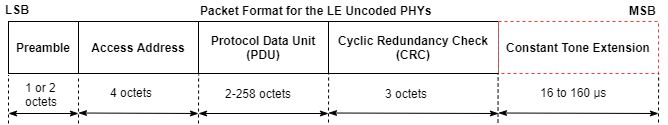Use the CTE in any of these communication types.

• Connection-oriented communication: It specifies the CTE using the new LL_CTE_RSP PDUs that are sent over the connection in response to the LL_CTE_REQ PDUs.

• Connectionless communication: It appends the CTE to the existing periodic advertising PDUs, AUX_SYNC_IND, for direction finding.

In connection-oriented and connectionless communication, the CTE is appended at the end of the PDU. For information about Bluetooth packet structures, see Bluetooth Packet Structure. For more information about the CTE, see volume 6, Part B, Section 2.5.1 of the Bluetooth Core Specification 5.1 .

### AoA and AoD Based Bluetooth LE Positioning

This example uses these terms:

• Bluetooth LE node - Specifies the device whose location is to be determined.

• Locator - Specifies the receiving device (in the AoA calculation) and transmitting device (in the AoD calculation).

This figure shows how to estimate the position of a Bluetooth LE node using the AoA and AoD methods.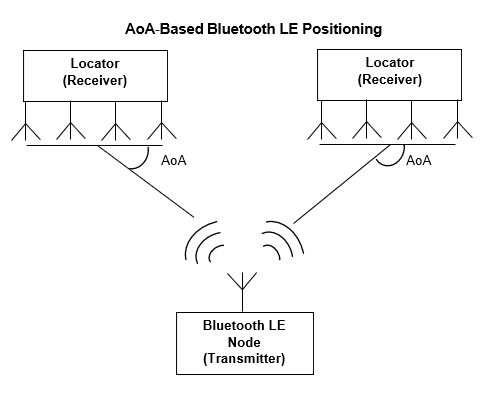In the AoA method, the transmitter (Bluetooth LE node) transmits a direction finding signal using single antenna. The receiving device (locator), equipped with an antenna array, takes the IQ samples while switching between the antennas present in the array. The locator uses the IQ samples to calculate the AoA.

In the AoD method, the transmitting device (locator) is equipped with an antenna array. The transmitting device transmits the signals while switching between the antennas in the array. The receiving device, consisting of a single antenna, collects the IQ samples and calculates the AoD.

To estimate the position of a Bluetooth LE node in 2-D or 3-D, the device requires at least two locators in the network, respectively. Based on the estimated angles and the known Bluetooth LE locator positions, estimate the position of a Bluetooth LE node using the triangulation technique.

### Simulation Parameters

Specify the dimension in which the Bluetooth LE node position needs to be determined and the number of Bluetooth LE locators. Specify at least two locators, to estimate the 2-D or 3-D position of a Bluetooth LE node.

```numDimensions =2; % Dimension of Bluetooth LE devices position in a network numLocators =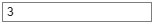3; % Number of locators```

Specify the Eb/No range and the number of iterations to simulate each Eb/No point.

```EbNo =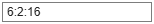6:2:16; % Eb/No in dB numIterations =200; % Number of iterations to average the position error```

Specify the direction finding method, the direction finding packet type, and the PHY transmission mode.

```dfMethod ="AoA"; % Direction finding method dfPacketType =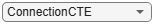"ConnectionCTE"; % Direction finding packet type phyMode ="LE1M"; % PHY transmission mode, must be LE1M or LE2M (for ConnectionCTE) and LE1M (for ConnectionlessCTE)```

Specify the antenna array parameters.

```arraySize =16; % Antenna array size, must be a scalar (represents ULA) or a row vector (represents URA) for 2D or 3D positioning, respectively elementSpacing =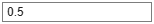0.5; % Normalized spacing between the antenna elements with respect to wavelength switchingPattern =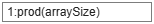1:prod(arraySize); % Antenna switching pattern, must be a 1xM row vector and M must be in the range [2, 74/slotDuration+1]```

Specify the waveform generation parameters.

```slotDuration =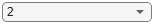2; % Slot duration in microseconds cteLength =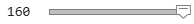160; % Length of CTE in microseconds, must be in the range [16, 160] with 8 microseconds step size sps =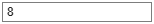8; % Samples per symbol channelIndex =17; % Channel index crcInit =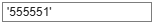'555551'; % CRC initialization accessAddress =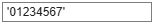'01234567'; % Access address payloadLength =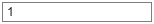1; % Payload length in bytes```

### Direction Finding and Position Estimation Procedure

Follow these steps to estimate the Bluetooth LE node position.

1. Position the Bluetooth LE node at the origin. Place the locators randomly in the 2-D or 3-D space.

2. Model the direction finding packet exchange between the Bluetooth LE node and each locator to estimate the angles between them.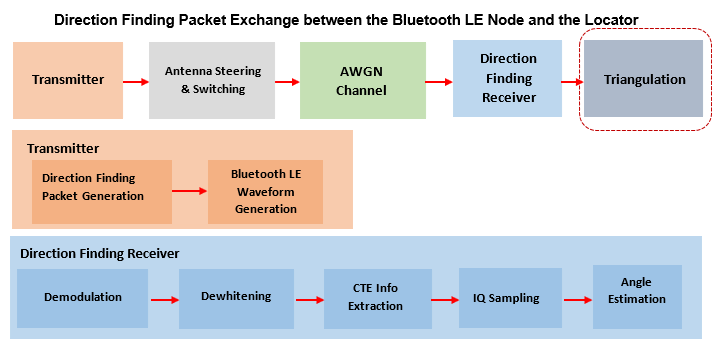a. Generate a direction finding packet for connection or connectionless communication.

b. Generate Bluetooth LE waveform.

c. Perform waveform steering and antenna switching.

d. Add AWGN to the waveform.

e. Perform demodulation, decoding, and IQ sampling on the noisy waveform.

f. Estimate the angle(s) between the Bluetooth LE node and each locator using the IQ samples.

3. Estimate the Bluetooth LE node position by performing triangulation using the known locator positions and the estimated angles.

For each iteration, assign random positions to the locators over a range of Eb/No points, and then repeat the preceding steps.

If you install Parallel Computing Toolbox™ to increase the speed of simulation, use a `parfor` loop by commenting-out the `for` statement and uncommenting the `parfor` statement in this code. The `parfor` loop processes each Eb/No point in parallel to reduce the total simulation time. If Parallel Computing Toolbox™ is not installed, by default the example switches to the `for` statement.

Validate the simulation parameters.

```minLocators = 2; % Minimum number of locators if numDimensions == 2 && size(arraySize,2) ~= 1 error('The arraySize must be a scalar for 2-D position estimation'); end if numDimensions == 3 && size(arraySize,2) ~= 2 error('The arraySize must be a 1-by-2 vector for 3-D position estimation'); end if numLocators < minLocators error(['The numLocators must be greater than or equal to ' num2str(minLocators)]); end if strcmp(dfPacketType,'ConnectionCTE') && payloadLength ~= 1 error('The payloadLength must be 1 byte for direction finding packet type of ConnectionCTE'); elseif strcmp(dfPacketType,'ConnectionlessCTE') && payloadLength < 3 error('The payloadLength must be greater than or equal to 3 bytes for direction finding packet type of ConnectionlessCTE'); end```

Create and configure Bluetooth LE angle estimation configuration object. Derive the type of CTE based on the slot duration and the direction finding method.

```if numDimensions == 3 && isscalar(elementSpacing) elementSpacing = [elementSpacing elementSpacing]; % Element spacing must be a vector to perform 3D positioning end cfg = bleAngleEstimateConfig('ArraySize',arraySize,'SlotDuration',slotDuration,'SwitchingPattern', ... switchingPattern,'ElementSpacing',elementSpacing); validateConfig(cfg); if strcmp(dfMethod,'AoA') cteType = [0;0]; else cteType = [0;1]; if slotDuration == 1 cteType = [1;0]; end end % Convert access address in hexadecimal to binary accessAddBits = int2bit(hex2dec(accessAddress),32,false); % Initialize the variables to be used outside the parfor loop numEbNo = numel(EbNo); % Number of Eb/No points posNode = zeros(numDimensions,numEbNo); posLocator = zeros(numDimensions,numLocators,numEbNo); angleEst = zeros(numLocators,numDimensions-1,numEbNo); posNodeEst = zeros(numDimensions,numEbNo); validResult = zeros(1,numEbNo); avgPositionError = zeros(1,numEbNo);```

Model the direction finding packet exchange between the Bluetooth LE node and each locator to estimate the angles between them.

```% parfor iEbNo = 1:numEbNo % Use 'parfor' to speed up the simulation for iEbNo = 1:numEbNo % Use 'for' to debug the simulation % Set the random substream index to ensure that each iteration uses a % repeatable set of random numbers stream = RandStream('combRecursive','Seed',12345); stream.Substream = iEbNo; RandStream.setGlobalStream(stream); % Loop over the number of iterations to average the positioning error. % If the successful links between the locators and node are less than % the minimum number of locators required to perform triangulation, then % the iteration fails. posErr = zeros(1,numIterations); iterationFailCount = 0; for iterCount = 1:numIterations % For each iteration, generate random positions for the locators [tempPosNode,tempPosLocator,ang] = helperBLEGeneratePositions(numLocators,numDimensions); posNode(:,iEbNo) = tempPosNode; posLocator(:,:,iEbNo) = tempPosLocator; % Loop over the number of locators tempAngleEst = zeros(numLocators,numDimensions-1); idx = []; linkFailFlag = zeros(numLocators,1); for i=1:numLocators % Generate direction finding packet data = helperBLEGenerateDFPDU(dfPacketType,cteLength,cteType,payloadLength,crcInit); % Generate Bluetooth LE waveform bleWaveform = bleWaveformGenerator(data,'Mode',phyMode,'SamplesPerSymbol',sps,... 'ChannelIndex',channelIndex,'DFPacketType',dfPacketType,'AccessAddress',accessAddBits); % Perform steering and switching between the antennas dfWaveform = helperBLESteerSwitchAntenna(bleWaveform,ang(i,:),... phyMode,sps,dfPacketType,payloadLength,cfg); % Pass the waveform through AWGN channel snr = EbNo(iEbNo) - 10*log10(sps); % Signal to noise ratio (SNR) noiseWaveform = awgn(dfWaveform,snr,'measured'); % Pass the noisy waveform to bleIdealReceiver which returns % the IQ samples [~, ~, iqSamples] = bleIdealReceiver(noiseWaveform,'Mode',phyMode,... 'SamplesPerSymbol',sps,'ChannelIndex',channelIndex,'DFPacketType',... dfPacketType,'SlotDuration',slotDuration); % Estimate the angle(s) using the IQ samples. A packet is % detected successfully when the minimum number of non-zero IQ % samples are present. refSampleLength = 8; % Reference samples length % IQ samples must contain at least eight samples from reference period and % one sample from each antenna minIQSamples = refSampleLength+getNumElements(cfg)-1; if length(nonzeros(iqSamples)) >= minIQSamples % If packet detection is successful tempAngleEst(i,:) = bleAngleEstimate(iqSamples,cfg); else linkFailFlag(i) = 1; % If packet detection fails, enable the link fail flag idx = [idx i]; %#ok<AGROW> % Store the indices corresponding to the locator-node communication fail links end end % If the successful node-locator links are greater than the minimum % number of locators and all the locator angles are not same, then % estimate the node position using triangulation and compute the % position error if (numLocators-nnz(linkFailFlag)) >= minLocators && ~isequal(tempAngleEst(:,1),repmat(tempAngleEst(1,1),numLocators,1)) % If any link fails, then assign NaN to the corresponding angle % estimates posLocatorEbNo = posLocator(:,:,iEbNo); tempAngEstTri = tempAngleEst; if any(linkFailFlag == 1) posLocatorEbNo(:,idx) = []; tempAngleEst(idx,:) = NaN(numel(idx),numDimensions-1); tempAngEstTri(idx,:) = []; end % Estimate the node position using triangulation posNodeEst(:,iEbNo) = blePositionEstimate(posLocatorEbNo,'angulation',tempAngEstTri.'); angleEst(:,:,iEbNo) = tempAngleEst; % Compute the position error posErr(iterCount) = sqrt(sum((posNodeEst(:,iEbNo)-posNode(:,iEbNo)).^2)); else iterationFailCount = iterationFailCount + 1; % Count the number of failed links used to average the position error end end if(iterationFailCount == numIterations) % If all the links fail at a given Eb/No value disp(['At Eb/No = ',num2str(EbNo(iEbNo)),' dB, all direction finding packet transmissions failed']) validResult(iEbNo) = 0; % Disable plot flag for failed links else avgPositionError(iEbNo) = sum(posErr)/(numIterations-iterationFailCount); disp(['At Eb/No = ',num2str(EbNo(iEbNo)),' dB, positioning error in meters = ', num2str(avgPositionError(iEbNo))]) validResult(iEbNo) = 1; % Enable plot flag for successful links end end```
```At Eb/No = 6 dB, positioning error in meters = 0.5594 At Eb/No = 8 dB, positioning error in meters = 0.43461 At Eb/No = 10 dB, positioning error in meters = 0.31708 At Eb/No = 12 dB, positioning error in meters = 0.27091 At Eb/No = 14 dB, positioning error in meters = 0.21464 At Eb/No = 16 dB, positioning error in meters = 0.17115 ```

### Simulation Results

If the direction finding packet transmission is successful, the example displays these plots.

• Bluetooth LE node position, locators positions, and the estimated node positions in 2-D or 3-D. The plot also shows the triangulation lines for the maximum Eb/No value and the last iteration.

• Average position error (in meters) versus Eb/No (in dB).

```[~,validIdx] = find(validResult==1); if ~isempty(validIdx) EbNoValid = EbNo(validIdx); [~,EbNoIdx] = max(EbNoValid); EbNoValidIdx = validIdx(EbNoIdx); helperBLEVisualizePosition(posLocator(:,:,EbNoValidIdx),posNode(:,EbNoValidIdx),... angleEst(:,:,EbNoValidIdx),posNodeEst(:,EbNoValidIdx)); figure plot(EbNoValid,avgPositionError(validIdx),'-b*','LineWidth',2, ... 'MarkerEdgeColor','b','MarkerSize',10) grid on xlabel('Eb/No (dB)') ylabel('Estimated Position Error (meters)') title('Position Accuracy in Bluetooth LE network') end```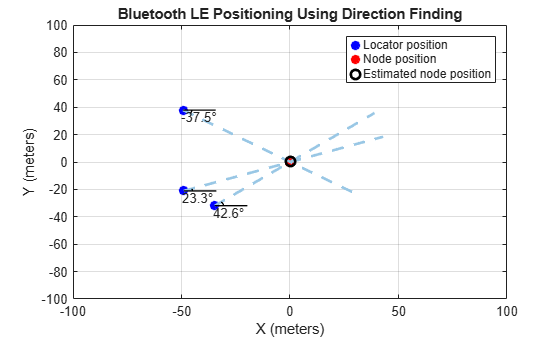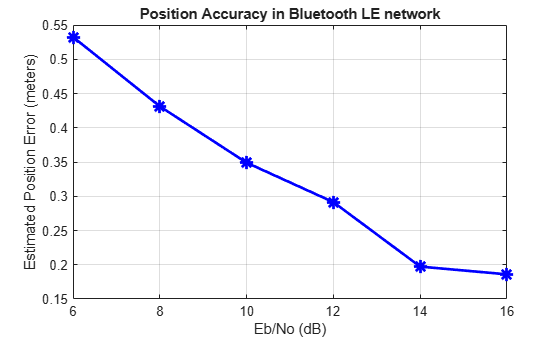This example enables you to estimate the 2-D or 3-D position of a Bluetooth LE node by using the Bluetooth LE direction finding functionality. The example shows how to implement the triangulation method to calculate the angles between the locators and the Bluetooth LE node. The example also shows how to measure the positioning accuracy related to the Eb/No value by computing the distance between the estimated and actual node positions in an AWGN channel.

### Appendix

The example uses these helpers:

### Selected Bibliography

1. Bluetooth Technology Website. “Bluetooth Technology Website | The Official Website of Bluetooth Technology.” Accessed November 22, 2021. https://www.bluetooth.com

2. Bluetooth Special Interest Group (SIG). "Core System Package [Low Energy Controller Volume]". Bluetooth Core Specification. Version 5.1, Volume https://www.bluetooth.com

3. Bluetooth Special Interest Group (SIG). "Core System Package [Low Energy Controller Volume]". Bluetooth Core Specification. Version 5.3, Volume https://www.bluetooth.com